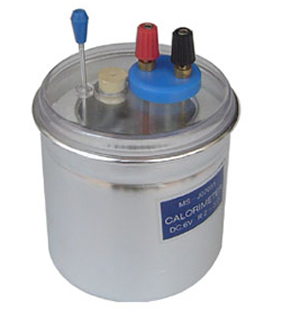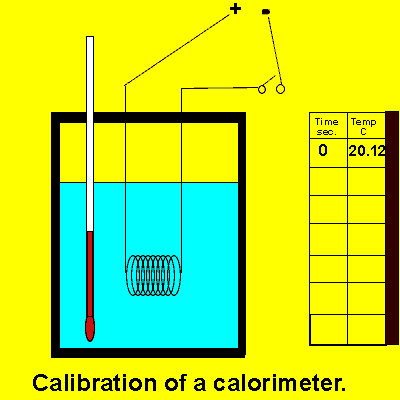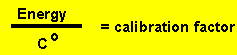The calorimeter The exact amount of energy released or absorbed during a chemical reaction is measured using an instrument known as a calorimeter. When a reaction occurs in a calorimeter the heat given off or absorbed, causes the temperature of the contents of the calorimeter to rise or fall. Before a calorimeter is of any use to us we must first calibrate it. Calibration of the calorimeter is the determination of how many joules of energy are required to raise the temperature of the contents by one degree Celsius. This is known as the calibration factor of the calorimeter. Since every calorimeter has unique differences, such as, thickness of insulating material and construction material which absorbs different amounts of heat, then the calorimeter will also have a unique calibration factor. Some clorimeters have a thick insulation padding while others are made of a wooden exterior..We calibrate the calorimeter by passing an electric current through a coil inside the calorimeter. We deliver a known amount of energy and measure the resultant rise in temperature. Energy is given by the formula E = VItwhere: "V" is voltage in volts "I" is current in amps "t" is time in seconds. The calibration factor is determined by the expression below.Consider the animation on the right. a) Calculate the energy, in joules, delivered to the contents of the calorimeter by the electrical coil. b) Calculate the calibration factor of the calorimeter. Solution